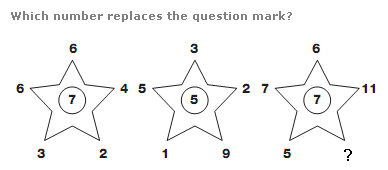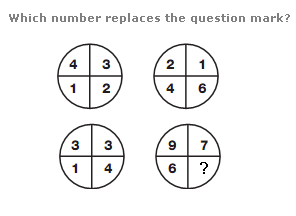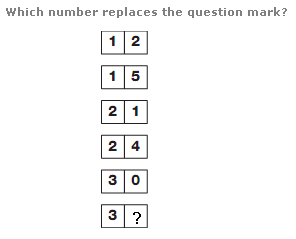# Puzzles - Number puzzles

### Exercise :: Number puzzlesAnswer : 6 Explanation : For each star, add the outer numbers together and divide by 3 for the left hand, 4 for the centre, and 5 for the right hand, putting the result in the centre.Answer : 12 Explanation : Add together numbers in corresponding segments of the upper two and lower left circles to give the values in corresponding segments of the lower right circle.Answer : 3 Explanation : Starting at the top and moving down, reading each pair of boxes as a 2 digit number, add the separate digits to the 2 digit number as a whole, and put the result in the 2 boxes below. Continue this sequence as you move down.